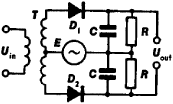# detector

(redirected from Toftness radiation detector)
Also found in: Dictionary, Thesaurus, Medical.

## detector:

see particle detectorparticle detector,
in physics, device for detecting, measuring, and analyzing particles and other forms of radiation entering it. Such devices play an important role not only in basic research, as in the study of elementary particles, but also in numerous applications of
.

## detector

The component in an instrument that is sensitive to the radiation or particles under observation. It may, for example, be an array of CCDs or a photographic plate. See also imaging.

## Detector

in radio engineering, a device for detecting electrical oscillations; used in broadcast, communications, and television receivers, measuring devices, and many other units to isolate the modulating frequencies of information-carrying oscillations. A distinction is made among amplitude detectors, frequency detectors, and phase discriminators.

In an amplitude detector, a semiconductor diode is most often used as a component with nonlinear electrical conductivity to detect high-frequency amplitude-modulated (AM) oscillations. The voltage of the AM oscillations, which is applied to an oscillatory circuit, acts on an electrical circuit that consists of a capacitor C, a diode D), and a resistor R that is connected either in series to a diode (series detector; Figure 1,a) or in parallel (parallel detector; Figure 1,b). As a result of the unilateral conductivity of the diode, an electrical current arises in the circuit in the form of high-frequency pulses whose amplitude changes according to the law of modulation of high-frequency oscillations. This current creates at the ends of resistor R a voltage whose amplitude also varies according to the law of modulation. For the high-frequency components of the current the electrical resistance of capacitor C is small, and consequently the voltage of the high-frequency oscillations at its ends is insignificant. For the modulating frequencies of the oscillations the voltage is much greater than the resistance of resistor R, and hence the voltage of the modulating frequencies of the oscillations is applied fully to the ends of resistor R.Figure 1. Circuitry of an amplitude detector with a semiconductor diode: (a) series, (b) parallel, (Lr) induction coil, (Cn) capacitor of oscillatory (resonance) circuit; (Uout) output voltage, (Rf) filter resistor, (Cf) filter capacitor, (D) semiconductor diode

An electrical filter that consists of a resistor Rf and a capacitor Cf is used to prevent the spurious capacitances of the electrical circuits connected to a parallel detector from affecting the high-frequency voltage fed to the diode. In most cases the detected voltage is then fed to an electrical signal amplifier. Transistors and electron tubes (triodes) are also used in amplitude detectors. A distinction is made among base, collector, emitter (or grid-lead), plate, and cathode detectors, depending on the electrode of the transistor or tube in the circuit to which the load is fed (resistor R).

Amplitude detection can also be accomplished by a linear change in time of the conductance of an electronic device (a diode or other component) in cycle with the carrier frequency of the received signal (a direct-conversion receiver). The conductance is changed by feeding to the input of the device the auxiliary oscillations (from the heterodyne) that have been synchronized by the carrier oscillations of the signal. A direct-conversion receiver has phase-selective properties; therefore, its use increases the noiseproofing of reception.

A single-cycle or two-cycle diode-based amplitude detector (to reduce nonlinear distortions of the signal) is used to detect unipolar AM oscillations. The received signal of the side band of frequencies and heterodyne oscillations with a frequency equal to the carrier frequency are fed to the detector input. Here the detector acts like a frequency converter.

In frequency detectors and phase discriminators, frequency-modulated and phase-modulated (FM and PM) oscillations are first converted into AM oscillations, which are then detected by an amplitude detector. In the simplest frequency detector, the oscillations are converted by an oscillatory circuit that is detuned with respect to the average frequency of the FM oscillations (see Figure l,a). When the detuning is slight the amplitude of the voltage taken from the circuit changes nearly in proportion to the detuning. There-fore, changes in the frequency of oscillations of an FM signal cause proportional changes in the amplitude of the oscillations in the circuit that are then fed to the diode (an amplitude detector). In a phase discriminator (Figure 2) the amplitude of the output depends on the phase shift between the received PM oscillations and the reference oscillations of the same frequency that are then fed to the input of an amplitude detector. A frequency detector with an additional electrical circuit that corrects for differences between the two types of modulation may be used to detect PM oscillations.Figure 2. Balanced circuit of phase discriminator: (T) transformer; (D1) and (D2) semiconductor diodes; (E) source of reference oscillations; (C) capacitor and (R) resistor, which together make up the load on the detector; (Uin) input voltage; (Uout) output voltage

### REFERENCES

Gutkin, L. S., V. L. Lebedev, and V. I. Siforov. Radiopriemnye ustroistva, part 1. Moscow, 1961.
Gonorovskii, I. S. Radiotekhnicheskie tsepi i signaly, part 2. Moscow, 1967.
Chistiakov, N. I., S. M. Khlytchiev, and O. M. Malochinskii. Radiosviaz’ i radioveshchanie, 2nd ed. Moscow, 1968.

IU. B. LIUBCHENKO

## detector

[di′tek·tər]
(electronics)
The stage in a receiver at which demodulation takes place; in a superheterodyne receiver this is called the second detector. Also known as demodulator; envelope detector.
(science and technology)
Apparatus or system used to detect the presence of an object, radiation, chemical compound, or such.

## sensor, detector, sensing device

A device which senses or detects an abnormal ambient condition, such as smoke or unusually high temperature; used to initiate an alarm signal, open a smoke hatch, etc.
Site: Follow: Share:
Open / Close